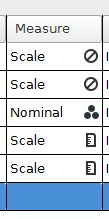# PSPP for Beginners

## Setting the Data Type

The measurement scale setting is particularly important because this informs PSPP about the scale of measurement or data type. This setting is worth some extra attention.There are three possible settings. Nominal scales represent categories. Our Sex variable is a category, so it was set to nominal. Ordinal scales (not shown above) are used for numbers that represent rankings. The Scale setting represents data that have equally sized units, such as seconds, grams, and meters. The Scale setting represents both interval scales (equal units but no zero point) and ratio scales (equal units and a zero point).

The measurement scales have helpful icons to assist in understanding their functions. The LastName and FirstName variables have a no symbol or prohibition sign. These are string variables, so numerical scale information is simply not relevant for these variables.For the symbols, nominal looks like a Venn diagram with no overlap between the circles. This is meant to represent the categories of a nominal variable. Ordinal looks like bar columns that progressively increase, thereby representing a ranking. Scale looks like a ruler with the equal intervals that a ruler contains.

The measurement scale setting can be useful when choosing the analyses at a later point in time. PSPP can use this information to help determine which tests might be the most appropriate for a given variable.